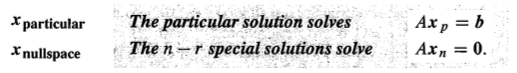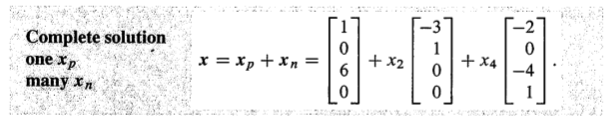Abstract: Ax=b的完整解，以及一个解，infinity个解，没有解的所有条件和说明
Keywords: Ax=b,Special Solution,Full Column Rank,Full Row Rank,Complete Solution

# 方程组的完整解

## 特解(Particular Solution)

$$\begin{bmatrix} 1&3&0&2\\ 0&0&1&4\\ 1&3&1&6 \end{bmatrix} \begin{bmatrix} x_1\\x_2\\x_3\\x_4 \end{bmatrix} = \begin{bmatrix} 1\\6\\7 \end{bmatrix}$$
Augmented Matrix:
$$\begin{bmatrix} 1&3&0&2&1\\ 0&0&1&4&6\\ 1&3&1&6&7 \end{bmatrix} = \begin{bmatrix} A&b \end{bmatrix}$$

$$\begin{bmatrix} 1&3&0&2&1\\ 0&0&1&4&6\\ 0&0&0&0&0 \end{bmatrix} = \begin{bmatrix} R&d \end{bmatrix}$$

$$\begin{bmatrix} x_1\\x_2\\x_3\\x_4 \end{bmatrix} = \begin{bmatrix} 1\\0\\6\\0 \end{bmatrix} =x_{particular}$$$$Ax_p=b\\ Ax_n=0\\ Ax_p+Ax_n=b+0\\ A(x_p+x_n)=b$$## 完整解(The Complete Solution)

1 r=m r=n Square and Invertible Ax=b 1
2 r=m r<n Short and Wide Ax=b $\infty$
3 r<m r=n Tall and Thin Ax=b 0 or 1
4 r<m r<n Not full rank Ax=b 0 or $\infty$

$$R=\begin{bmatrix}I\end{bmatrix}$$

$$R=\begin{bmatrix}I&F\end{bmatrix}$$

$$R=\begin{bmatrix}I\\0\end{bmatrix}$$

$$R=\begin{bmatrix}I&F\\0&0\end{bmatrix}$$

0%